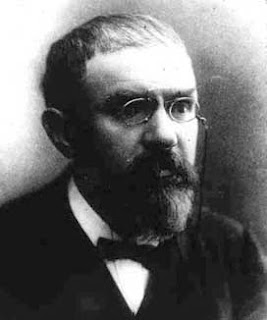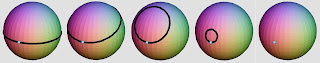### The Poincaré conjecture"It is by logic that we prove, but by intuition that we discover. To know how to criticize is good, to know how to create is better" — H.Poincaré, Science and Method (1908, Part II. Ch. 2, p. 129)

The Poincaré Conjecture is a result in mathematics which we have taken as the main subject of our piece. It is a very important and useful result concerning shape and dimension, and has only recently been proven, having been stated over a hundred years ago.

Henri Poincaré (1854-1912) was a French mathematician of extraordinary breadth, depth, and ability. He made original fundamental contributions to many fields, including celestial mechanics, differential equations, complex function theory, algebraic geometry, topology, number theory, celestial mechanics, electromagnetic theory, and the theory of relativity.

He was a prolific writer and creative thinker who revolutionised the mathematics of his day. What is now known as the Poincaré conjecture was first posed as a question in a paper written in 1904:

If a three-dimensional shape (compact three-manifold) is simply connected, is it homeomorphic to the three-sphere?

Understanding this question takes a little more information - firstly, when he mentions a 'three-dimensional shape', this is not in the sense you might be used to. A shape like a sphere, or a flat piece of paper, is mathematically two-dimensional - in that you can describe a point on its surface using two coordinates. If you are standing on the surface of it, you can move in two dimensions.

Three-dimensional objects, like the ones Poincaré is interested in, have three directions you can move in at any point - and they exist in four-dimensional space. This means that, since the universe we live in has three spatial dimensions, the Poincaré conjecture might be able to tell us something about what shape it is.

The word 'homeomorphic' is a mathematical term which means that something is fundamentally the same - and one can be stretched or bent into the shape of the other, without cutting or gluing. For example, a square is homeomorphic to a circle, but a sphere is not homeomorphic to something with a hole in it, such as a doughnut.If we stretch a rubber band around the surface of an apple, then we can shrink it down to a point by moving it slowly, without tearing it and without allowing it to leave the surface. On the other hand, if we imagine that the same rubber band has somehow been stretched in the appropriate direction around a doughnut, then there is no way of shrinking it to a point without breaking either the rubber band or the doughnut. We say the surface of the apple is "simply connected," but that the surface of the doughnut is not. Poincaré, almost a hundred years ago, knew that a two-dimensional sphere is essentially characterised by this property of simple connectivity, and asked the corresponding question for the three dimensional sphere (the set of points in four dimensional space at unit distance from the origin).
This question turned out to be extraordinarily difficult. Nearly a century passed between its formulation in 1904 by Henri Poincaré and its solution by Grigori Perelman, announced in 2002. Perelman's solution was based on Richard Hamlton's theory of Ricci flow, and made use of several other existing results on spaces by Perelman himself and other mathematicians. In these papers Perelman also proved William Thurston's Geometrization Conjecure, a special case of which is the Poincaré conjecture.

The Poincaré Conjecture is famous for another reason: it is one of the eight Millennium Mathematics Problems selected by the Clay Institute, and as such its solution, suitably verified, attracts a million dollar prize. However, Perelman had his own reasons for not wanting the prize - indeed, any reward save the solution itself. He turned down the prize, making the solution of the conjecture even more of a news story and attracting much media attention, and in 2006 he also declined the award of a Fields Medal for his work.